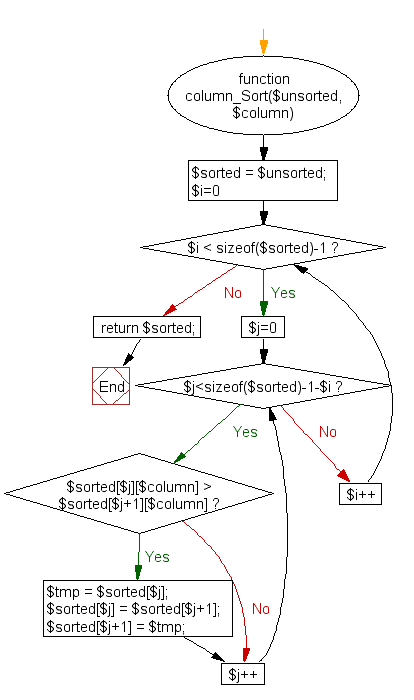﻿ PHP Array Exercise: Sort a multi-dimensionall array set by a specific key - w3resource# PHP Array Exercises : Sort a multi-dimensional array set by a specific key

## PHP Array: Exercise-23 with Solution

Write a PHP program to sort a multi-dimensional array set by a specific key.

Sample Solution:

PHP Code:

``````<?php
function column_Sort(\$unsorted, \$column) {
\$sorted = \$unsorted;
for (\$i=0; \$i < sizeof(\$sorted)-1; \$i++) {
for (\$j=0; \$j<sizeof(\$sorted)-1-\$i; \$j++)
if (\$sorted[\$j][\$column] > \$sorted[\$j+1][\$column]) {
\$tmp = \$sorted[\$j];
\$sorted[\$j] = \$sorted[\$j+1];
\$sorted[\$j+1] = \$tmp;
}
}
return \$sorted;
}
\$my_array = array();
\$my_array['name'] = 'Sana';
\$my_array['email'] = '[email protected]';
\$my_array['phone'] = '111-111-1234';
\$my_array['country'] = 'USA';

\$my_array['name'] = 'Robin';
\$my_array['email'] = '[email protected]';
\$my_array['phone'] = '222-222-1235';
\$my_array['country'] = 'UK';

\$my_array['name'] = 'Sofia';
\$my_array['email'] = '[email protected]';
\$my_array['phone'] = '333-333-1236';
\$my_array['country'] = 'India';
print_r(column_Sort(\$my_array, 'name'));
?>
```
```

Sample Output:

```Array
(
 => Array
(
[name] => Robin
[email] => [email protected]
[phone] => 222-222-1235
[country] => UK
)

 => Array
(
[name] => Sana
[email] => [email protected]
[phone] => 111-111-1234
[country] => USA
)

 => Array
(
[name] => Sofia
[email] => [email protected]
[phone] => 333-333-1236
[country] => India
)

)
```

Flowchart:PHP Code Editor:

What is the difficulty level of this exercise?

Test your Programming skills with w3resource's quiz.

﻿

## PHP: Tips of the Day

In PHP, there are two versions of logical AND and OR operators.

Operator True if
\$a and \$b Both \$a and \$b are true
\$a && \$b Both \$a and \$b are true
\$a or \$b Either \$a or \$b is true
\$a || \$b Either \$a or \$b is true

Note that the && and || opererators have higher precedence than and and or. See table below:

Evaluation Result of \$e Evaluated as
\$e = false || true True \$e = (false || true)
\$e = false or true False (\$e = false) or true

Because of this it's safer to use && and || instead of and and or.